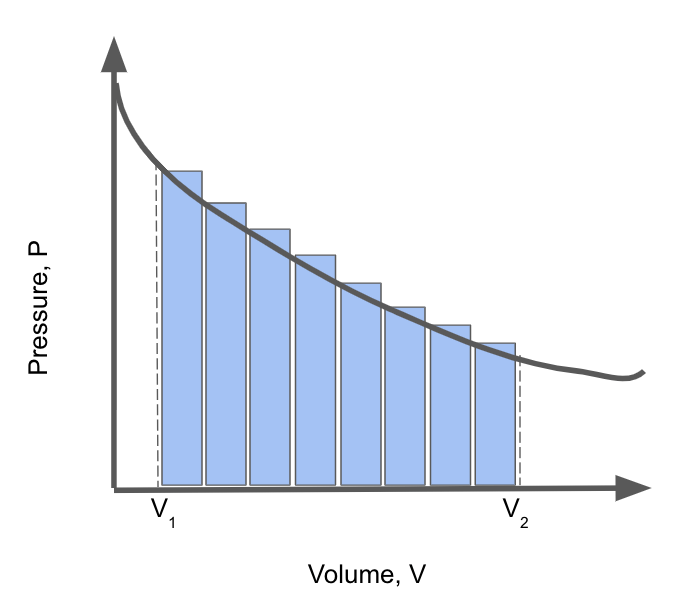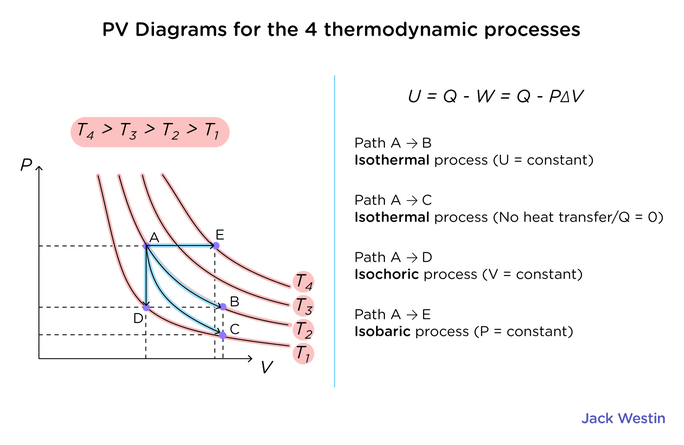Cyber Sale - Only 3 Days Remaining: ALL MCAT Products & Admissions Services

MCAT Content / Energy Changes In Chemical Reactions / Pv Diagram Work Done Area Under Or Enclosed By Curve

### PV Diagram: Work Done = Area Under or Enclosed by Curve

Topic: Energy Changes In Chemical Reactions

Pressure-Volume (PV) Diagrams are used to describe the pressure and volume relationship in a dynamic(changing) system. The area under the curve in a PV diagram describes the work done in the system.From the pressure vs. volume diagram above, it can be seen that the increase in volume results in a decrease in pressure. An important piece of data that can be taken from this diagram is the amount of work done by the system. This value can be taken by taking the integral of the function between V1 and V2. In other words, the area under the curve can be calculated to find the amount of work done. From this graph, we can derive that if the final volume is greater than the initial volume, more work is done; the max work that can be done in a system in regard to pressure and volume is a system that can have reversible gas expansion.

Practice Questions

MCAT Official Prep (AAMC)

Official Guide C/P Section Question 27

Key Points

• The work done by a system can be calculated by the area under a curve in a pressure-volume diagram.

• A reversible process can produce the greatest amount of work.

Key Terms

work: form of energy transfer in a system

reversible: process where the system can be returned to its original state

Billing Information